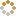﻿ N-B-U-T Online Judge ::  Countless Core Computers
• ###  Countless Core Computers

• 时间限制: 3000 ms　内存限制: 65535 K
• 问题描述
• The computer is greater and greater now.

You can imagine that a computer with countless cores. That will be Greeeeeat!

So the computer can deal with tasks continuous but not cut into small ones.

Give you N tasks and its start time and end time. Each core can only deal with one task at a moment and the tasks should not be cut into small ones. The problem will ask you several questions that how many cores are at work at moment T.

• 输入
• This problem contains several cases. Input until EOF.
The first line of each case contains an integer N (1 <= N <= 500000), stand for the number of tasks.
Then follows N lines, each line stands for a task. It contains the ith task's start time and end time. (0 <= starttime <= endtime <= 5000000).
Then it will be an integer Q (1 <= Q <= 500000), stands for the number of questions.
Next Q lines, each line is an integer q (0 <= q <= max (endtime)), stands for the moment that you're asked.
• 输出
• For each question, you should output how many cores are at work at that moment. Each question per line.
• 样例输入
• ```3
1 3
2 4
3 5
3
2
3
4
```
• 样例输出
• ```2
3
2
```
• 提示
• `无`
• 来源
• `XadillaX`
• 操作
﻿